## RD Sharma Class 10 Solutions Chapter 3 Pair of Linear Equations in Two Variables Ex 3.4

These Solutions are part of RD Sharma Class 10 Solutions. Here we have given RD Sharma Class 10 Solutions Chapter 3 Pair of Linear Equations in Two Variables Ex 3.4

Other Exercises

Solve each of the following systems of equations by the method of cross multiplication.
Question 1.
x + 2y + 1 = 0
2x – 3y – 12 = 0 (C.B.S.E. 1992)
Solution: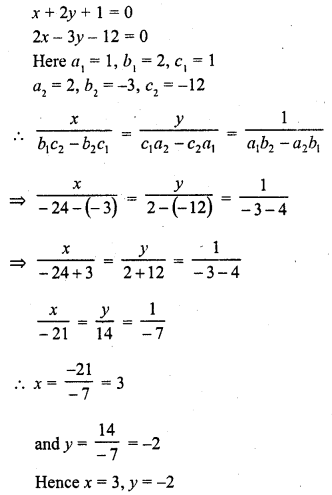Question 2.
3x + 2y + 25 = 0
2x + y + 10 = 0 (C.B.S.E. 1992)
Solution: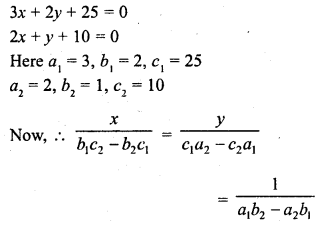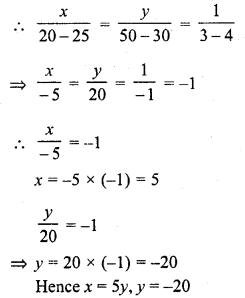Question 3.
2x + y = 35
3x + 4y = 65 (C.B.S.E. 1993)
Solution: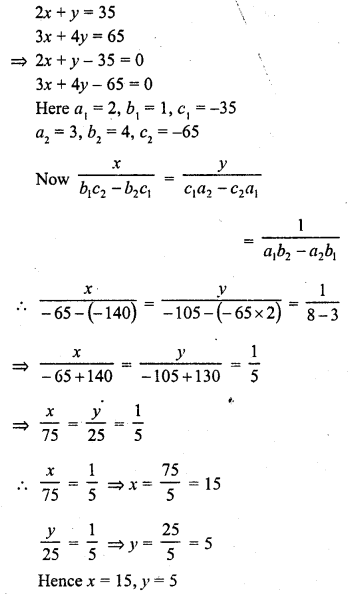Question 4.
2x – y = 6
x – y = 2 (C.B.S.E. 1994)
Solution:Question 5.
$$\frac { x+y }{ xy } =2,\quad \frac { x-y }{ xy } =6$$
Solution: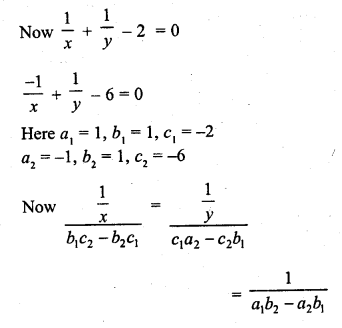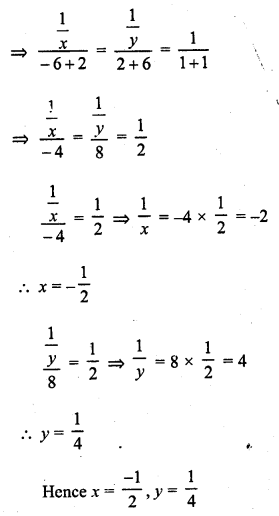Question 6.
ax + by = a – b
bx – ay = a + b
Solution:
ax + by = a – b
bx – ay = a + b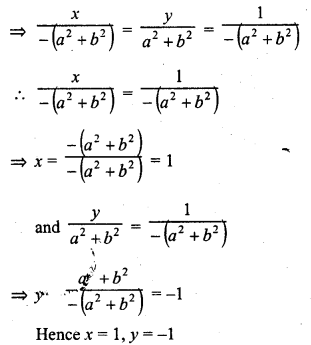Question 7.
x + ay = b
ax – by = c
Solution:
x + ay = b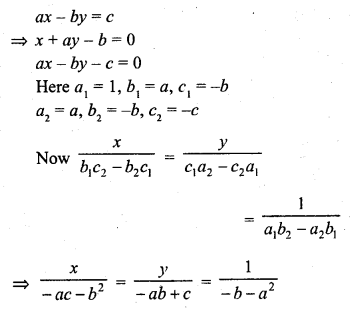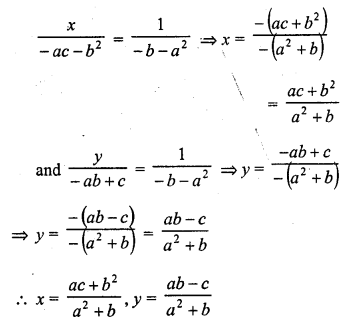Question 8.
ax + by = a2
bx + ay = b2
Solution: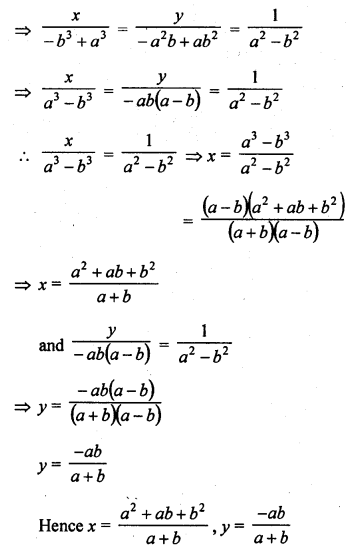Question 9.Solution:Question 10.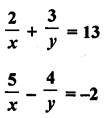Solution: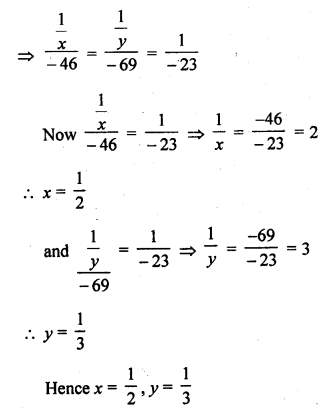Question 11.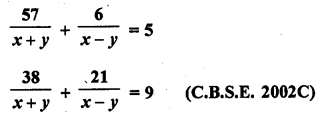Solution: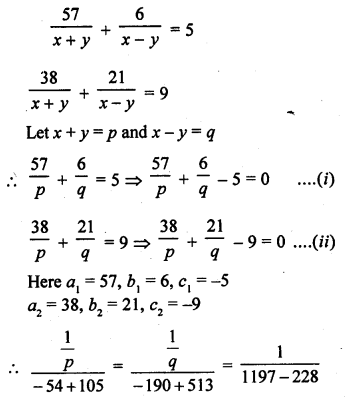Question 12.Solution:Question 13.
$$\frac { x }{ { a } } +\frac { y }{ b } =a+b$$
$$\frac { x }{ { { a }^{ 2 } } } +\frac { y }{ { b }^{ 2 } } =2$$
Solution: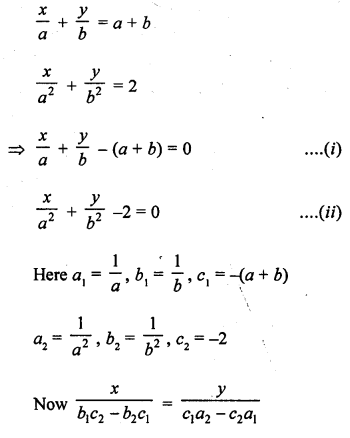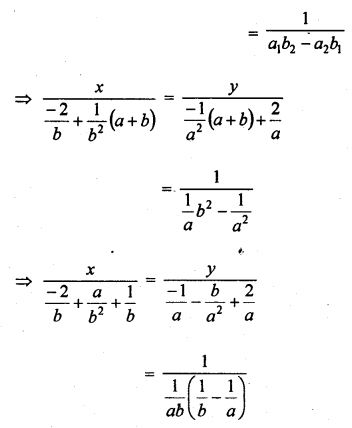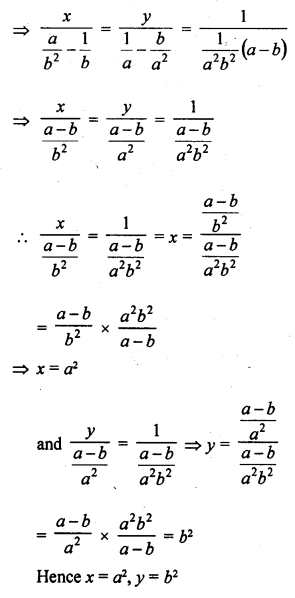Question 14.
$$\frac { x }{ { { a } } } =\frac { y }{ { b } }$$
ax + by = a2 + b2
Solution: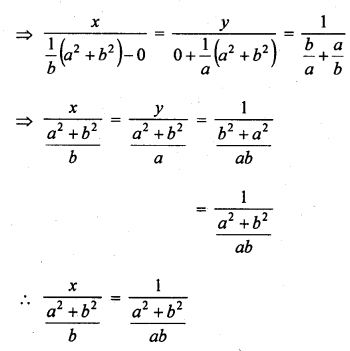Question 15.
2ax + 3by = a + 2b
3ax + 2by = 2a + b
Solution: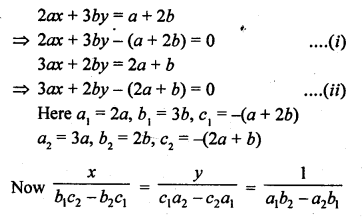Question 16.
5ax + 6by = 28
3ax + 4by = 18
Solution: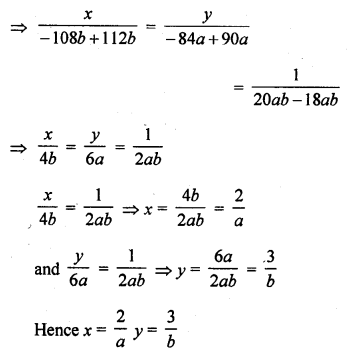Question 17.
(a + 2b) x + (2a – b) y = 2
(a – 2b) x + (2a + b) y = 3
Solution: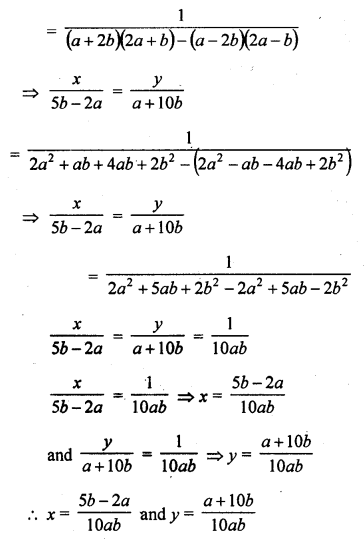Question 18.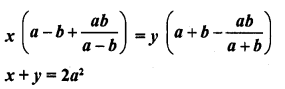Solution: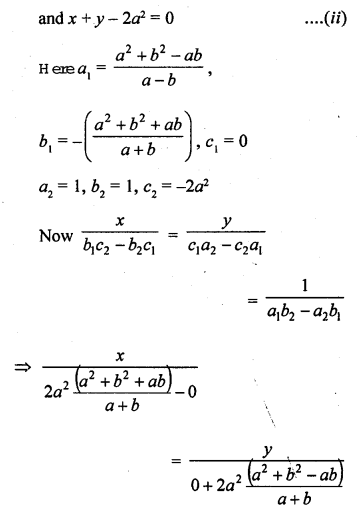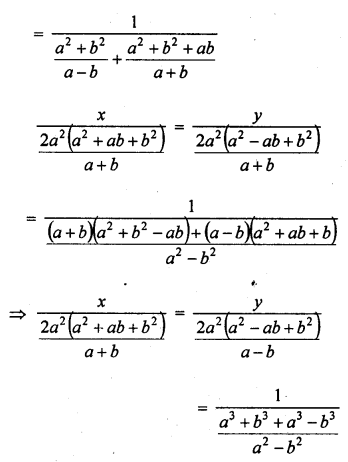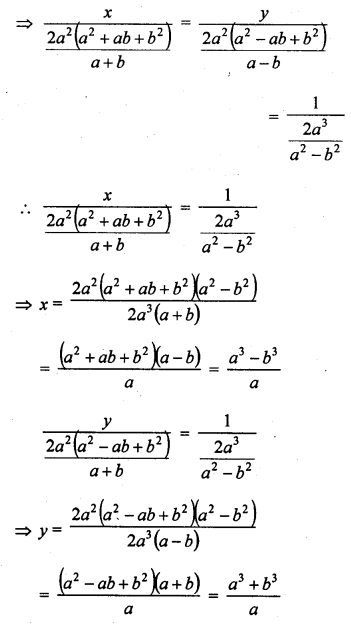Question 19.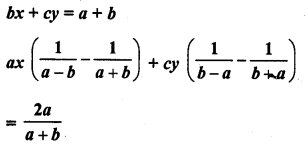Solution: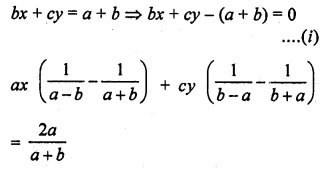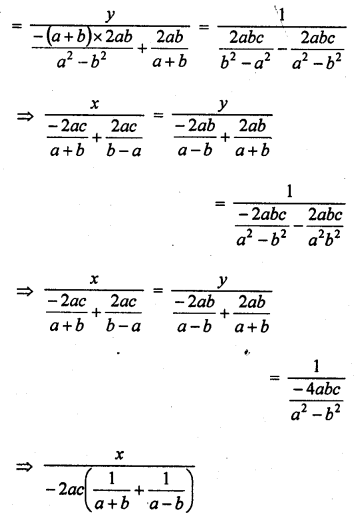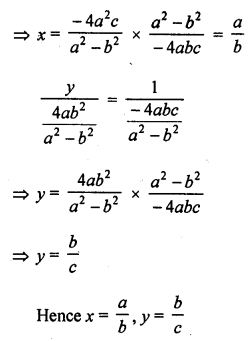Question 20.
(a – b) x + (a + b) y = 2a2 – 2b2
(a + b) (x + y) = 4ab
Solution: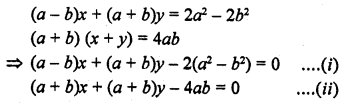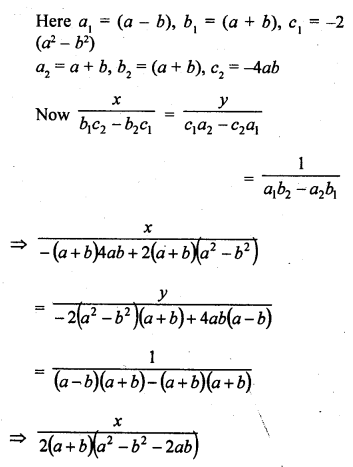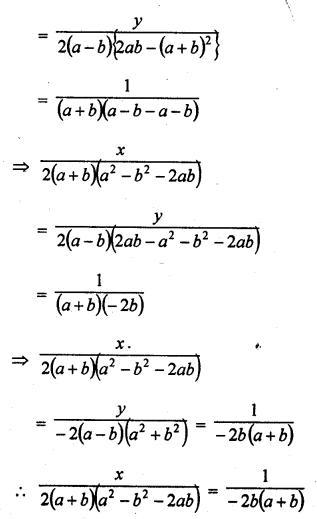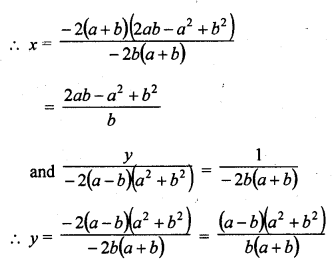Question 21.
a2x + b2y = c2
b2x + a2y = d2
Solution: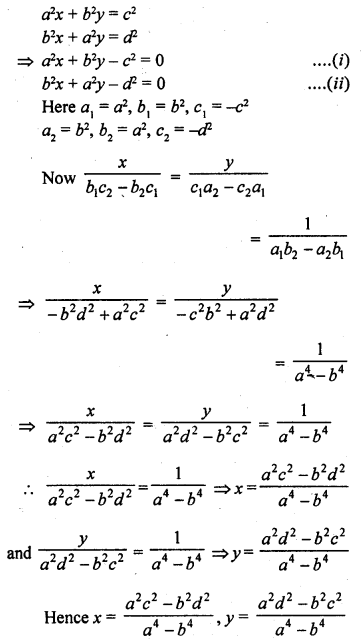Question 22.
ax + by = $$\frac { a + b }{ 2 }$$
3x + 5y = 4
Solution:Hence x = $$\frac { 1 }{ 2 }$$ , y = $$\frac { 1 }{ 2 }$$

Question 23.
2 (ax – by) + a + 4b = 0
2 (bx + ay) + b – 4a = 0 (C.B.S.E. 2004)
Solution: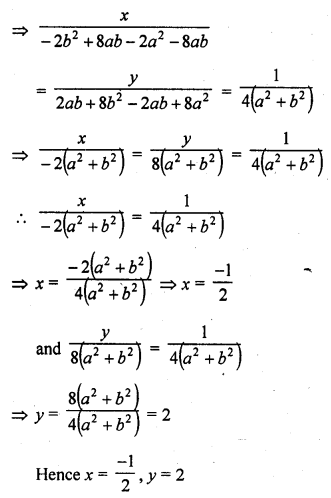Question 24.
6 (ax + by) = 3a + 2b
6 (bx – ay) = 3b – 2a (C.B.S.E. 2004)
Solution: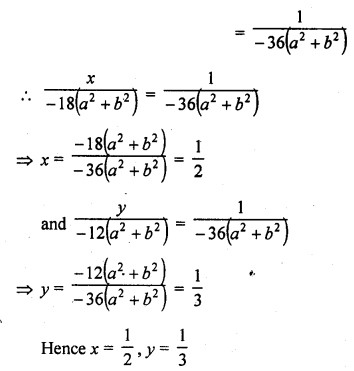Question 25.
$$\frac { { a }^{ 2 } }{ x } -\frac { { b }^{ 2 } }{ y } =0$$
$$\frac { { a }^{ 2 }b }{ x } -\frac { { b }^{ 2 }a }{ y } =a+b\quad ,\quad x,y\quad \neq 0$$
Solution: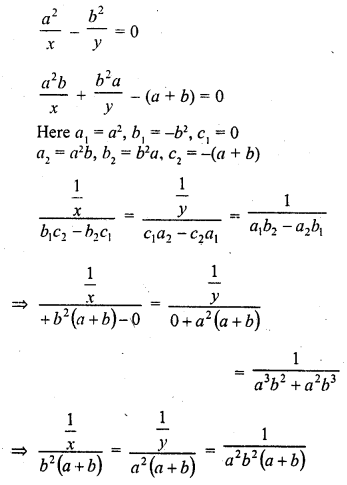Question 26.
mx – ny = m2 + n2
x + y = 2m (C.B.S.E. 2006C)
Solution: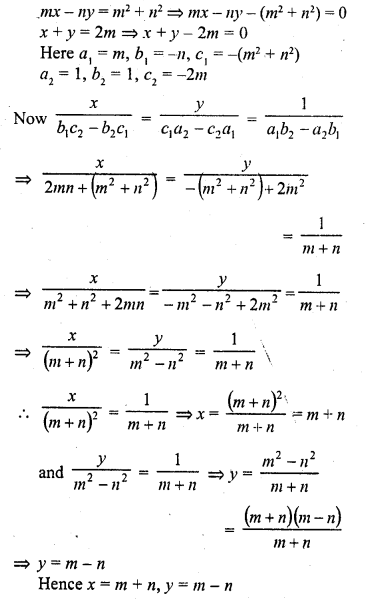Question 27.Solution: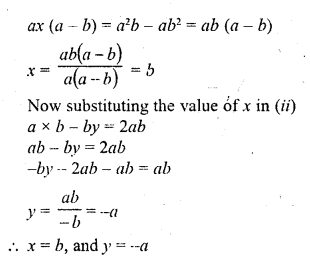Question 28.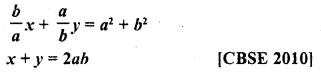Solution: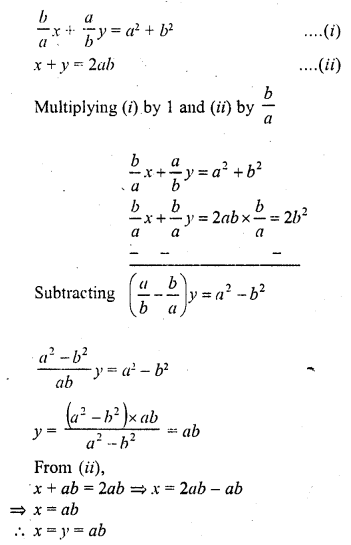Hope given RD Sharma Class 10 Solutions Chapter 3 Pair of Linear Equations in Two Variables Ex 3.4 are helpful to complete your math homework.

If you have any doubts, please comment below. Learn Insta try to provide online math tutoring for you.